# Second-Order Estimator Verification Guide

In this post I provide some tools for the do-it-yourself CSP practitioner. One of the goals of this blog is to help new CSP researchers and students to write their own estimators and algorithms. This post contains some spectral correlation function and cyclic autocorrelation function estimates and numerically evaluated formulas that can be compared to those produced by anybody’s code.

The signal of interest is, of course, our rectangular-pulse BPSK signal with symbol rate$0.1$ (normalized frequency units) and carrier offset$0.05$. You can download a MATLAB script for creating such a signal here.

The formula for the SCF for a textbook BPSK signal is published in several places (The Literature [R47], My Papers ) and depends mainly on the Fourier transform of the pulse function used by the textbook signal.

We’ll compare the numerically evaluated spectral correlation formula with estimates produced by my version of the frequency-smoothing method (FSM). The FSM estimates and the theoretical functions are contained in a MATLAB mat file here. (I had to change the extension of the mat file from .mat to .doc to allow posting it to WordPress.) In all the results shown here and that you can download, the processed data-block length is$65536$ samples and the FSM smoothing width is$0.02$ Hz. A rectangular smoothing window is used. For all cycle frequencies except zero (non-conjugate), a zero-padding factor of two is used in the FSM.

For the cyclic autocorrelation, we provide estimates using two methods: inverse Fourier transformation of the spectral correlation estimate and direct averaging of the second-order lag product in the time domain.

### Power Spectrum

Let’s look at the PSD first. The graph below shows a plot of the theoretical PSD for the signal plus noise and the corresponding FSM estimate. The signal power is one and the noise power is$1/10$. The mainlobe and the two adjacent sidelobes match nearly perfectly; there is a small discrepancy three or four sidelobes away from the mainlobe. Overall, I judge this an excellent match between theory and measurement.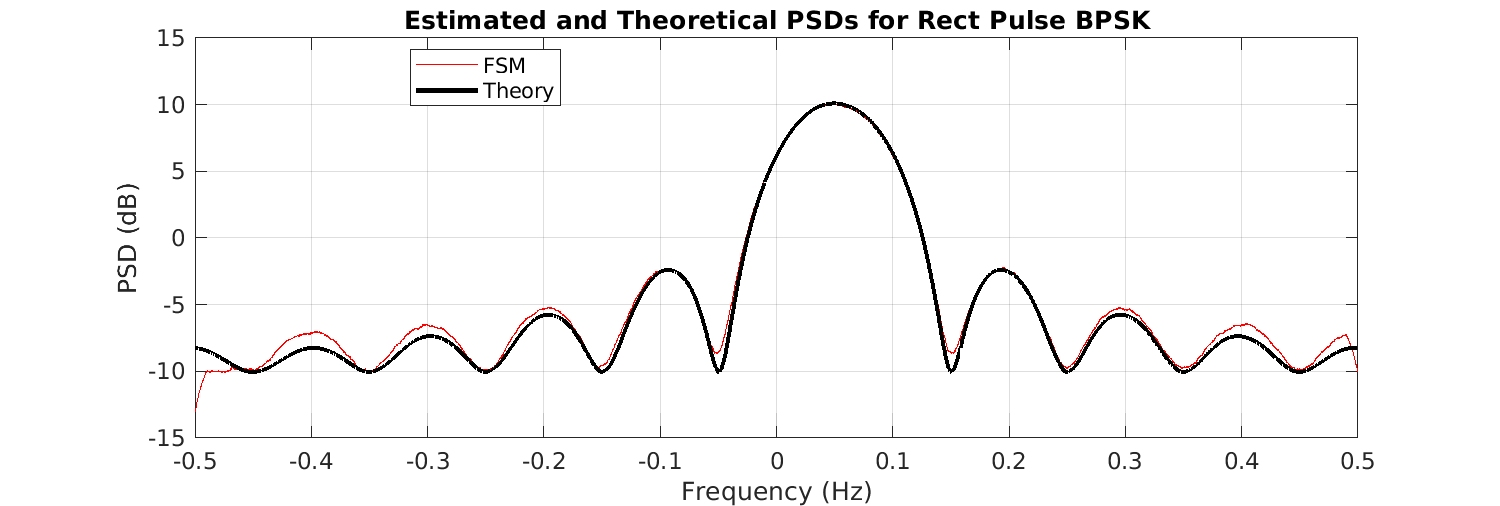### Non-Conjugate Spectral Correlation

Next consider the non-conjugate cycle frequencies, which are harmonics of the bit rate$0.1$. The first three harmonics are$0.1, 0.2$, and$0.3$, and the theoretical and FSM-estimated non-conjugate spectral correlation function magnitudes are shown in the following graph:### Conjugate Spectral Correlation

The following graph shows three of the conjugate spectral correlation function magnitudes. The conjugate cycle frequencies are equal to the doubled carrier plus harmonics of the BPSK bit rate, or$\alpha = 2f_c + k/T_0 = 0.1 + 0.1k$. The three functions shown below correspond to$k = -1, 0, 1$.### Non-Conjugate Cyclic Autocorrelation

Each of the functions above is inverse Fourier transformed and the resulting cyclic autocorrelation estimates are plotted: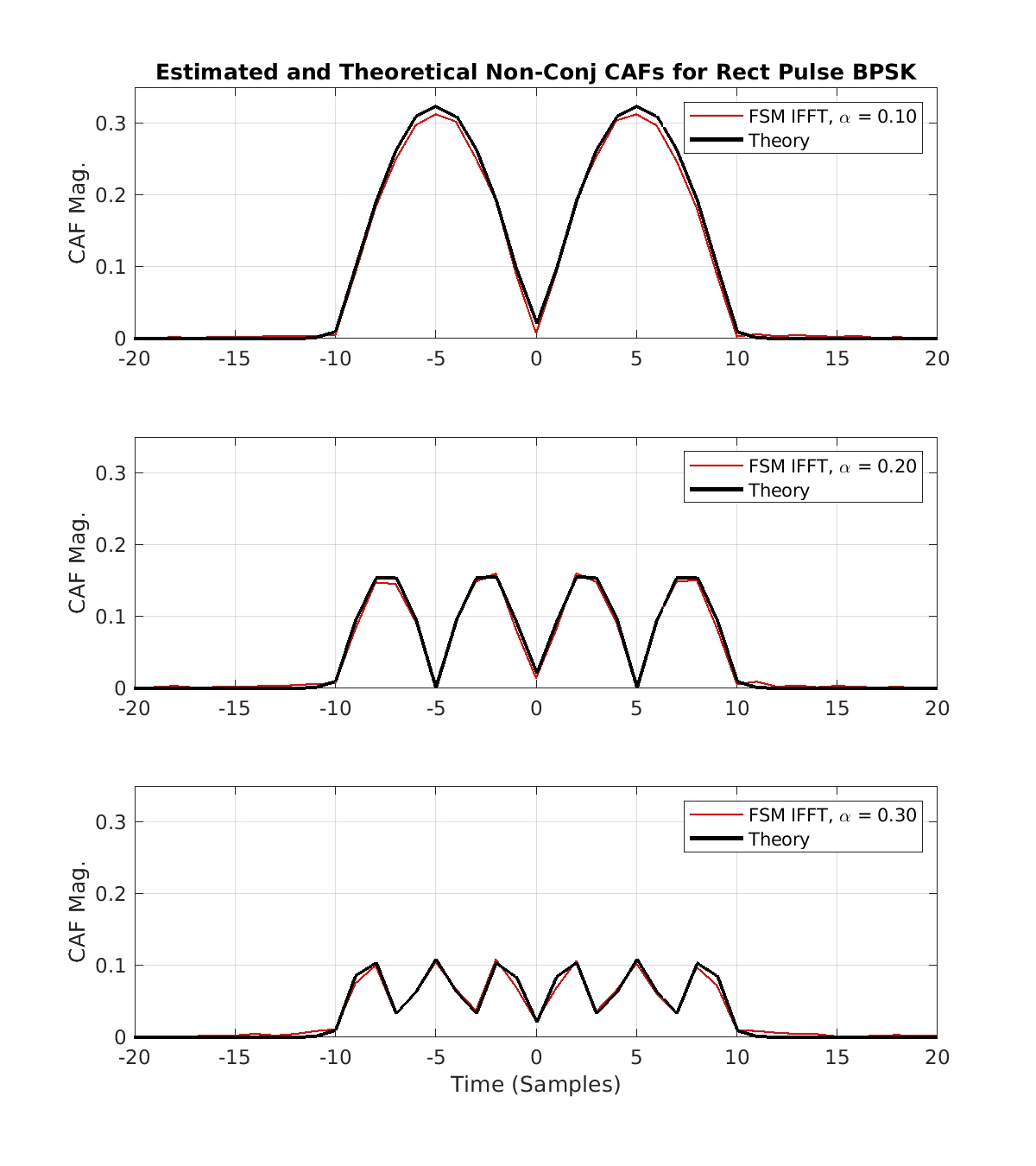The cyclic autocorrelation functions are also directly estimated in the time domain by simply implementing the defining average: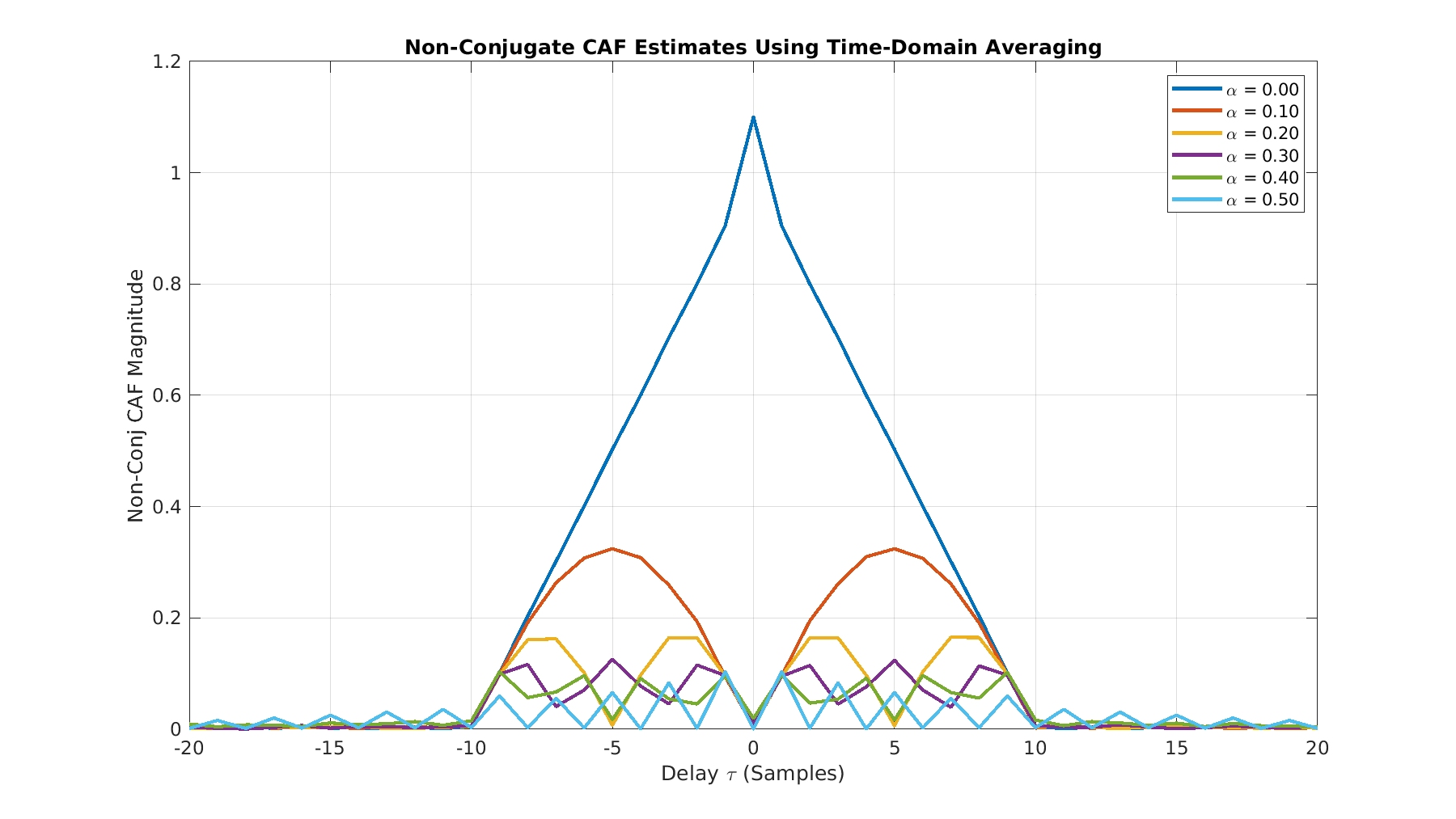### Conjugate Cyclic Autocorrelation

The conjugate cyclic autocorrelation functions are estimated by inverse Fourier transforming the FSM spectral correlation estimates and by direct time-domain averaging of the lag product: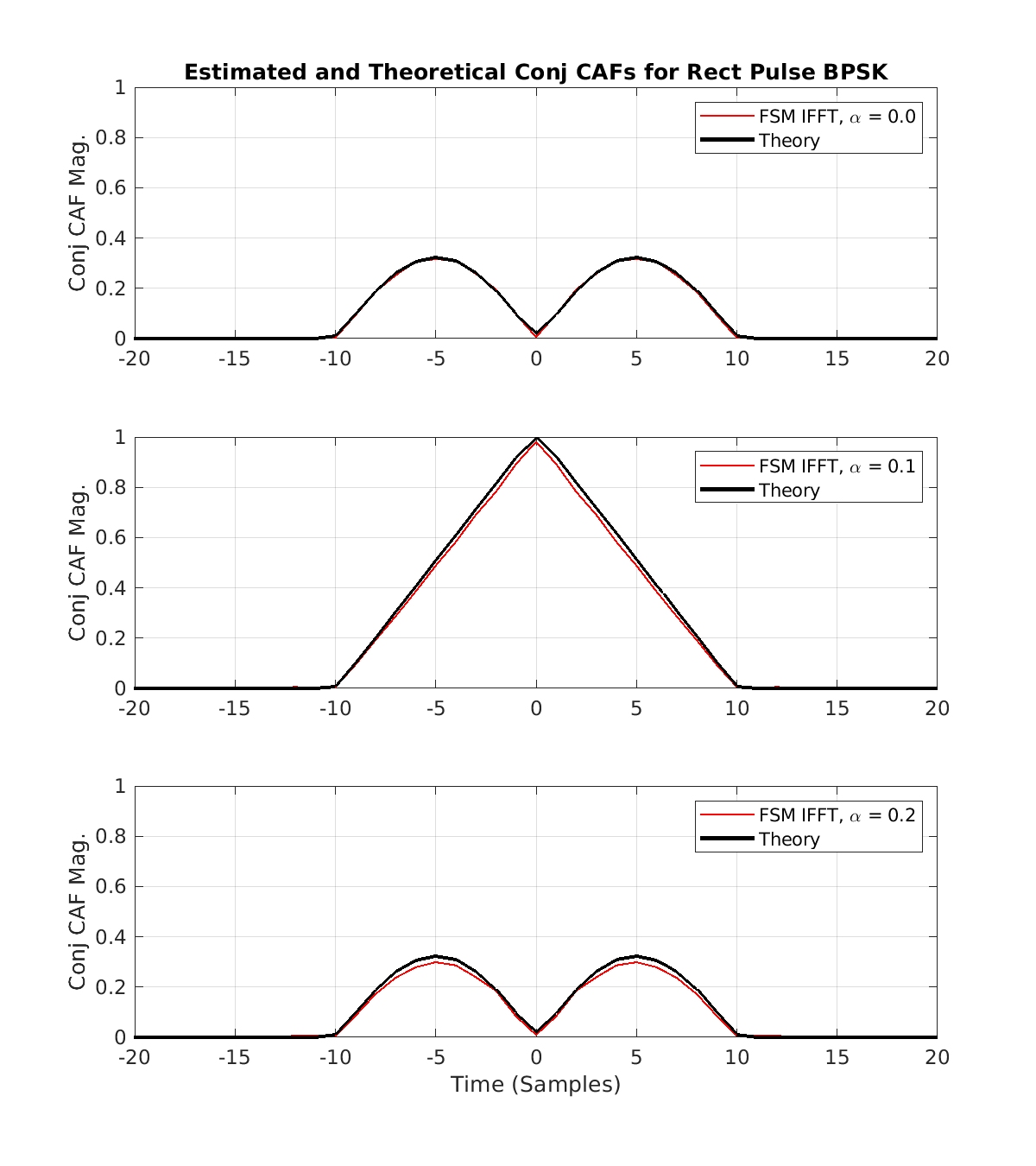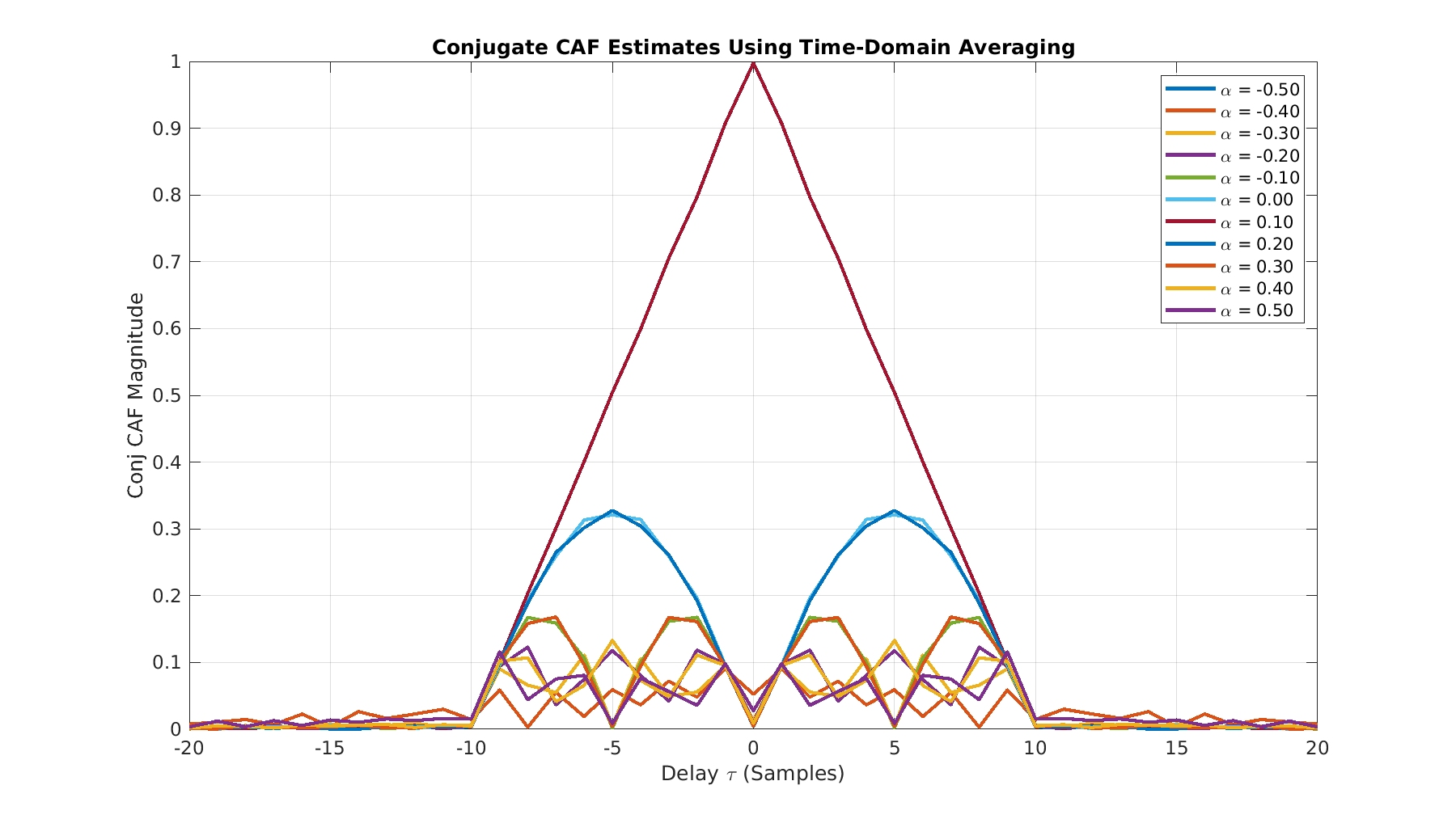The matches between theory and measurement are excellent. This should give you confidence in comparing the results here with the output of your estimator when you use the rectangular-pulse BPSK signal.

Don’t forget to download the MATLAB file containing all the functions plotted in this post (including their time or frequency vectors that define the x-axis) here.I'm a signal processing researcher specializing in cyclostationary signal processing (CSP) for communication signals. I hope to use this blog to help others with their cyclo-projects and to learn more about how CSP is being used and extended worldwide.

Posted on

## 3 thoughts on “Second-Order Estimator Verification Guide”

1.Lelele Viyviy says:

I can’t open theory_and_meas_functions_mat file. How can I succeed it?

1.Chad Spooner says:

The file is a .mat file, but I renamed it with a .doc filename extension so that WordPress software would allow me to upload it to the blog. Rename the file from theory_and_meas_functions_mat.doc to theory_and_meas_functions.mat and then use ‘load’ in MATLAB. Let me know if that doesn’t work for you, and if it does not, exactly what errors you see.

2.ytachwali says:

Chad, thank you for this wonderful blog.

For python users, they can use loadmat() function from scipy.io:
https://docs.scipy.org/doc/scipy/reference/tutorial/io.html

However, for certain Matlab versions (including the one used to create theory_and_meas_functions.mat), I was able to open it with hdf5 reader. Here is a sample code to do so:
 import h5py f = h5py.File('/my_file_location/theory_and_meas_functions_mat.mat') for item in f.keys():    dataset=f[item]    print(dataset) f.close()